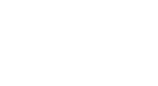# Client Lab Supply Order Form

Rady Children's Hospital Laboratory Department   For questions call: (858) 576-1700 ext. 5629
Requests received by 12 midnight Monday will deliver Wednesday.
IMPORTANT: Please make sure to properly submit your order by clicking the "Submit Order" button at the bottom of this page.
1. Please enter your contact information below:
(Start typing then select name from pop-up choices OR type in if not listed)
This question requires a valid email address.
Please include Mailcodes (MC) in this field.
This question requires a valid number format.
Packages (100 units/pk)
This question requires a valid number format.
Packages (100 units/pk)
This question requires a valid number format.
Packages (100 units/pk)
This question requires a valid number format.
Each (1 unit/ea)
This question requires a valid number format.
Each (1 unit/ea)
This question requires a valid number format.
Each (1 unit/ea)
This question requires a valid number format.
Boxes (50 units/box)
This question requires a valid number format.
Boxes (50 units/box)
This question requires a valid number format.
Each (1 unit/each)
This question requires a valid number format.
Each (1 unit/each)
This question requires a valid number format.
Each (1 unit/ea)
This question requires a valid number format.
Each (1 unit/ea)
This question requires a valid number format.
Each (1 unit/ea)
This question requires a valid number format.
Bags (50 units/BG)
This question requires a valid number format.
Each (1 unit/ea)
This question requires a valid number format.
Each (1 unit/ea)
This question requires a valid number format.
Each (1 unit/ea)
This question requires a valid number format.
Each (1 unit/ea)
This question requires a valid number format.
Each (1 unit/ea)
This question requires a valid number format.
Bags (50 units/BG)
This question requires a valid number format.
Bags (50 units/BG)
This question requires a valid number format.
Packs (100 units/PK)
This question requires a valid number format.
Each (1 unit/ea)
This question requires a valid number format.
Each (1 unit/ea)
This question requires a valid number format.
Boxes (100 units/box)
This question requires a valid number format.
Boxes (100 units/box)
This question requires a valid number format.
Boxes (100 units/box)
This question requires a valid number format.
Boxes (100 units/box)
 SCM TO OBTAIN FROM LAB
This question requires a valid number format.
Each (1 unit/ea)
This question requires a valid number format.
Each (1 unit/ea)
This question requires a valid number format.
Each (100 unit minimum)
This question requires a valid number format.
Each (1 unit/ea)
This question requires a valid number format.
Each (1 unit/ea)
This question requires a valid number format.
Each (1 unit/ea)
This question requires a valid number format.
Each (1 unit/ea)
IMPORTANT! Please make sure to click the "Submit Order" button only once, and do not close the browser or navigate away from this page until the form is finished submitting (you will see a Thank You page and receive an email receipt of your order when properly submitted). All required field errors must be corrected for your order to be processed.